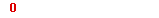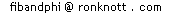# The Fibonacci series

is formed by starting with 0 and 1 and then adding the latest two numbers to get the next one:
```
0 1 --the series starts like this.
0+1=1 so the series is now
0 1 1
1+1=2 so the series continues...
0 1 1 2 and the next term is
1+2=3 so we now have
0 1 1 2 3  and it continues as follows ...
```On these pages, the first Fibonacci number is 1, as is the second, the third being 2, the fourth 3 and so on. We will often include Fib(0)=0 too:

 n: Fib(n): 0 1 2 3 4 5 6 7 8 9 10 11 12 13 14 15 16 ... 0 1 1 2 3 5 8 13 21 34 55 89 144 233 377 610 987 ..More..© 1996-2007 Dr Ron Knottupdated November 2003